# Simulate FMCW Interference Between Automotive Radars

This example shows how to simulate interference between two FMCW automotive radars in a highway scenario. Missed and ghost detections caused by interferences can be simulated and visualized. Multipath propagation effects between vehicles are taken into account.

### Introduction

With the increasing number of vehicles being equipped with multiple radars in automotive scenarios, interference detection and mitigation have become essential for automotive radars. Interference can affect the functionality of radar systems in many different ways. Interference can increase the noise level of the CFAR detection algorithm and decrease the probability of detection. The signal to interference and noise ratio (SINR) depends on the distance and angles separating two radars, as well as radar parameters such as antenna gain, antenna transmit power, bandwidth, center frequency, and chirp duration. Low SINR results in missed detections for low RCS targets, creating a blind spot in some regions.

Another challenge with radar interference is ghost targets, in which the radar detects an object that doesn't exist. It may happen when the victim radar and interfering radar have similar waveforms. The victim radar can mitigate the probability of false alarms caused by ghost targets by changing the waveform timing parameters on a frame-to-frame basis. The false-alarm probability can also be mitigated by a tracking algorithm on the victim's radar.

### Define Victim FMCW Radar Waveform

The victim radar is designed to use an FMCW waveform which is a common waveform in automotive applications because it enables range and Doppler estimation through computationally efficient FFT operations. For illustration purposes, in this example, configure the radar to a maximum range of 150 m. The victim radar operates at a 77 GHz frequency. The radar is required to resolve objects that are at least 1 meter apart. Because this is a forward-facing radar application, the radar also needs to handle targets with large closing speeds as high as 230 km/h.

```rng(2017); % Set random number generator for repeatable results fc = 77e9; % Center frequency (Hz) c = physconst('LightSpeed'); % Speed of light (m/s) lambda = freq2wavelen(fc,c); % Wavelength (m) rangeMax = 150; % Maximum range (m) rangeRes = 1; % Desired range resolution (m) vMax = 230*1000/3600; % Maximum velocity of cars (m/s) fmcwwav1 = generateFMCWWaveform(fc,rangeMax,rangeRes,vMax); sig = fmcwwav1(); Ns = numel(sig);```

### Define Interfering FMCW Radar Waveform

Consider an interfering radar using FMCW modulation. The frequency spectrum characteristics of the interference in the victim radar depend on the chirp's relative timing and slopes.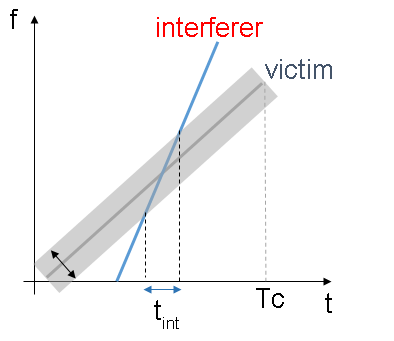```fcRdr2 = 77e9; % Center frequency (Hz) lambdaRdr2 = freq2wavelen(fcRdr2,c); % Wavelength (m) rangeMaxRdr2 = 100; % Maximum range (m) rangeResRdr2 = 0.8; % Desired range resolution (m) vMaxRdr2 = vMax; % Maximum Velocity of cars (m/s) fmcwwav2 = generateFMCWWaveform(fcRdr2,rangeMaxRdr2,rangeResRdr2,vMaxRdr2); sigRdr2 = fmcwwav2();```

```tiledlayout(1,2) pspectrum(repmat(sig,3,1),fmcwwav1.SampleRate,'spectrogram', ... 'Reassign',true,'FrequencyResolution',10e6) axis([0 15 -80 80]); title('Victim Radar'); colorbar off nexttile```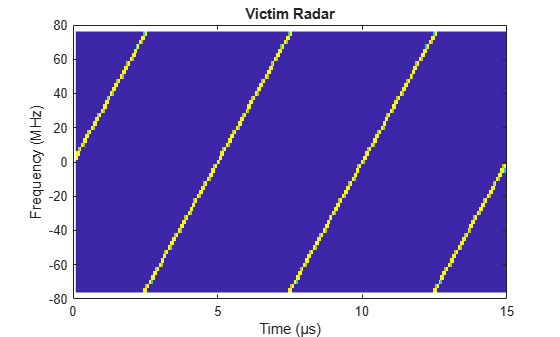```pspectrum(repmat(sigRdr2,3,1),fmcwwav1.SampleRate,'spectrogram',... 'Reassign',true,'FrequencyResolution',10e6,'MinThreshold',-13) axis([0 15 -80 80]); title('Interfering Radar'); colorbar off;```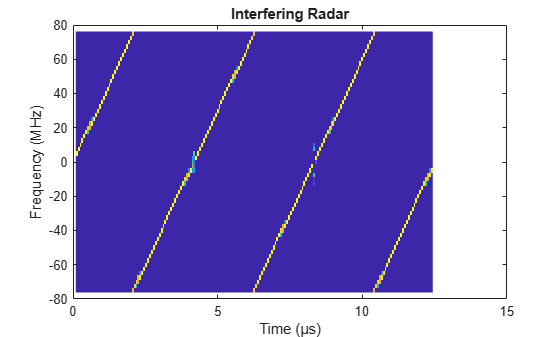### Model Victim and Interfering Radar Transceivers

Consider both radars use an isotropic element to transmit and a uniform linear array (ULA) to receive the radar waveforms. Using a linear array enables the radar to estimate the azimuthal direction of the reflected energy received from the target vehicles. The long-range radar needs to detect targets across a coverage area of 45 degrees in front of the ego vehicle.

```% Construct the array for Victim Radar Nve = 2*3; % Number of virtual antenna with 2Tx, 3Rx antElmnt = phased.IsotropicAntennaElement('BackBaffled',false); rxVArray = phased.ULA('Element',antElmnt,'NumElements',Nve,'ElementSpacing',freq2wavelen(fc)/2,'Taper',taylorwin(Nve)); antAperture = 6.06e-4; % Antenna aperture (m^2) antGain = aperture2gain(antAperture,lambda); % Antenna gain (dB) txPkPower = db2pow(13)*1e-3; % Tx peak power (W) rxNF = 4.5; % Receiver noise figure (dB) % Model Victim Radar Transceiver vradar = radarTransceiver("Waveform",fmcwwav1); vradar.Transmitter = phased.Transmitter('PeakPower',txPkPower,'Gain',antGain); vradar.TransmitAntenna = phased.Radiator('Sensor',antElmnt,'OperatingFrequency',fc); vradar.ReceiveAntenna = phased.Collector('Sensor',rxVArray,'OperatingFrequency',fc); vradar.Receiver = phased.ReceiverPreamp('Gain',antGain,'NoiseFigure',rxNF,'SampleRate',fmcwwav1.SampleRate); % Define Victim Radar VictimRadar = radarTransceiver('Waveform',fmcwwav1,'Transmitter',vradar.Transmitter, ... 'TransmitAntenna',vradar.TransmitAntenna,'ReceiveAntenna',vradar.ReceiveAntenna ,'Receiver',vradar.Receiver); ```

Model the transceiver of the interfering radar as a clone of the victim radar using different FMCW parameters.

```% Model Interfering Radar Transceiver iradar = clone(vradar); iradar.Waveform = fmcwwav2; vradar.Receiver = phased.ReceiverPreamp('Gain',antGain,'NoiseMethod','Noise power','NoisePower',0);```

### Define Range Doppler Response

The radar collects multiple sweeps of the waveform on each of the linear phased array antenna elements. These collected sweeps form a data cube, which is defined in Radar Data Cube. These sweeps are coherently processed along the fast- and slow-time dimensions of the data cube to estimate the range and Doppler of the vehicles.

Use the `phased.RangeDopplerResponse` object to perform the range and Doppler processing on the radar data cubes. Use a Hann window to suppress the large sidelobes produced by the vehicles when they are close to the radar.

```Nft = fmcwwav1.SweepTime*fmcwwav1.SampleRate; % Number of fast-time samples Nsweep = 192; % Number of slow-time samples Nr = 2^nextpow2(Nft); % Number of range samples Nd = 2^nextpow2(Nsweep); % Number of Doppler samples rngdopresp = phased.RangeDopplerResponse('RangeMethod','FFT', ... 'DopplerOutput','Speed','SweepSlope',fmcwwav1.SweepBandwidth/fmcwwav1.SweepTime, ... 'RangeFFTLengthSource','Property','RangeFFTLength',Nr, ... 'RangeWindow','Hann', ... 'DopplerFFTLengthSource','Property','DopplerFFTLength',Nd, ... 'DopplerWindow','Hann', ... 'PropagationSpeed',c,'OperatingFrequency',fc,'SampleRate',fmcwwav1.SampleRate);```

### Model Free Space Propagation Channel for Interference

Use the free space channel to model the propagation of the transmitted and received signals for the interfering radar. In a free space model, the radar energy propagates along a direct line-of-sight between radars and the target vehicles.

```% Create a multipath channel for interfering radar channelIradar = helperCreateMultiPathChannel('SampleRate',fmcwwav2.SampleRate, ... 'OperatingFrequency',fcRdr2);```

### Simulate Driving Scenario

Create a highway driving scenario with two vehicles traveling in the vicinity of the ego vehicle. The vehicles are modeled as cuboids and have different velocities and positions defined in the driving scenario. The ego vehicle is moving with a velocity of 25 m/s and the other two vehicles are both moving at 50 m/s in the opposite direction. For details on modeling a driving scenario see the example Create Actor and Vehicle Trajectories Programmatically (Automated Driving Toolbox). The radar sensor is mounted on the front of the ego and interferer vehicles.

To create the driving scenario, use the helperAutoDrivingScenario function. To examine the contents of this function, use the `edit('helperAutoDrivingScenario')` command.

```% Create driving scenario [scenario, egoCar] = helperAutoDrivingScenario;```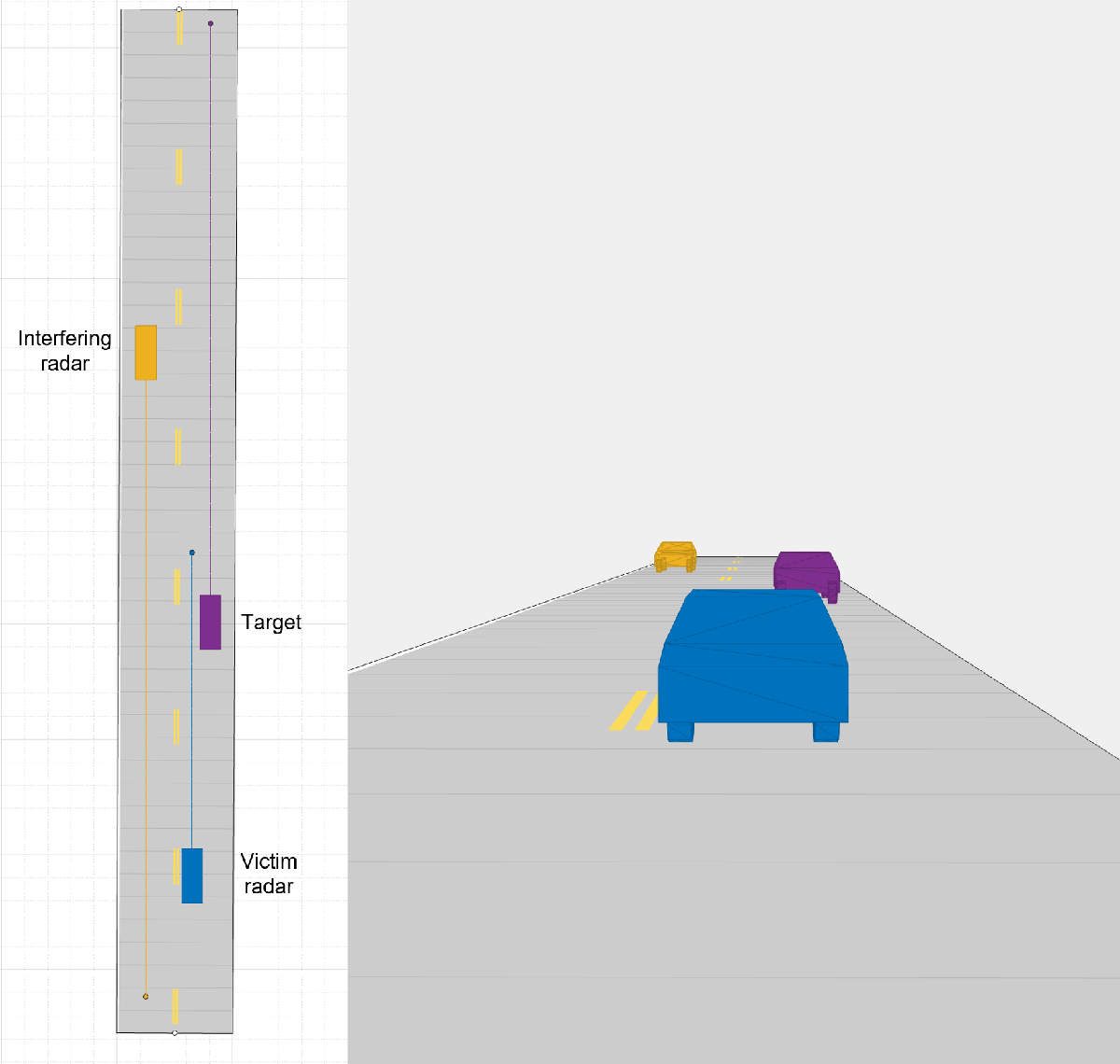The following loop uses the drivingScenario object to advance the vehicles in the scenario. A radar data cube is assembled at every simulation time step by collecting 192 sweeps of the radar waveform. The assembled data cube is then processed in range and Doppler.

```% Run the simulation loop sweepTime = fmcwwav1.SweepTime; while advance(scenario) % Get the current scenario time time = scenario.SimulationTime; % Get current target poses in ego vehicle's reference frame tgtPoses = targetPoses(egoCar); actProf = actorProfiles(scenario); % Assemble data cube at current scenario time Xcube = zeros(Nft,Nve,Nsweep); XcubeVradar = zeros(Nft,Nve,Nsweep); % Generate waveform signal to transmit for interfering radar sIsig = step(iradar.Waveform); % Match the length of interfering signal with victim sweep sIsig = repmat(sIsig,ceil(Nft/length(sIsig)),1); for m = 1:Nsweep sIcar = sIsig(1:Nft); sIsig = circshift(sIsig,length(sIsig)-Nft); % Generate all paths to victim radar [vpaths, ipaths] = helperGeneratePaths(tgtPoses,actProf,[lambda lambdaRdr2]); % Global to local coord system(s) inside % Send signal from interfering radar to power amplifier for transmit IcarSig = step(iradar.Transmitter,sIcar); % Radiate signal from antenna elements of interfering radar xtIcar = step(iradar.TransmitAntenna,IcarSig,[ ipaths(1).AngleOfDeparture ipaths(2).AngleOfDeparture]); % Propagate signal along paths [xrxIradar,angIradar ]= step(channelIradar, xtIcar, ipaths,time); % Pass the signals through the receive antenna array elements xcIradar = step(vradar.ReceiveAntenna, xrxIradar, angIradar); % Pass the signals at the output of the receive antenna element to the receiver amplifier rxInt = step(vradar.Receiver,xcIradar); % Get the received signal without interference rxVictim = VictimRadar(vpaths,time); % Dechirp the received signal rxVsig = dechirp(rxVictim,sig); % Save sweep to data cube - create ADC data XcubeVradar(:,:,m) = rxVsig; % Dechirp the received signal + Interference and save sweep to data cube rx = dechirp(rxVictim+rxInt,sig); Xcube(:,:,m) = rx; % Get the current scenario time time = time + fmcwwav1.SweepTime; % Move targets forward in time for next sweep tgtPoses(1).Position=[tgtPoses(1).Position]+[tgtPoses(1).Velocity]*fmcwwav1.SweepTime; tgtPoses(2).Position=[tgtPoses(2).Position]+[tgtPoses(2).Velocity]*fmcwwav1.SweepTime; end % Calculate the range-Doppler response [Xrngdop,rnggrid,dopgrid] = rngdopresp(Xcube); % Plot Range-Doppler Map figure surf(dopgrid, rnggrid,((20*log10(abs(squeeze(mean(Xrngdop,2))))))) zlabel('Power, dB'); xlabel('Velocity, m/s'); ylabel('Range, m') title('RDM','FontSize',12); colormap(jet); colorbar; axis square; shading interp view([-10,55]); clim([-100 30]); axis([-120 120 0 100 -77 50]) % Calculate the range-Doppler response with NO Interference and plot the RDM [XrngdopVradar,rnggrid,dopgrid] = rngdopresp(XcubeVradar); % Plot Range-Doppler Map figure surf(dopgrid, rnggrid,((20*log10(abs(squeeze(mean(XrngdopVradar,2))))))) zlabel('Power, dB'); xlabel('Velocity, m/s'); ylabel('Range, m') title('RDM, No Interference','FontSize',12) colormap(jet); colorbar; axis square; shading interp view([-10,55]); clim([-100 30]); axis([-120 120 0 100 -77 50]) break end```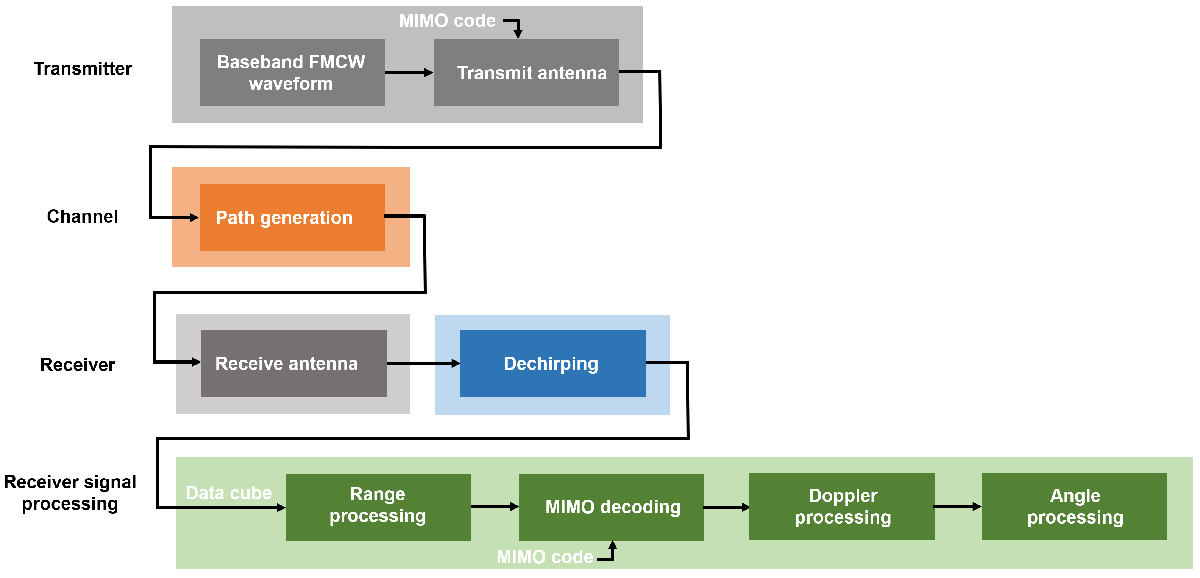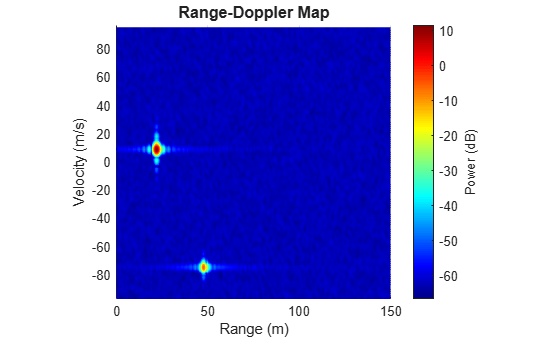The following animation shows the range-Doppler map for the victim radar for the duration of this scenario.The following figure shows the interference effects for FMCW radars with different waveforms for the interfereing radar. In this example, the chirp time for the victim radar is considered to be 5$\mu \mathit{s}$, and the chirp time for the interfering radar is changed from 1.6$\mu \mathit{s}\text{\hspace{0.17em}}$to 5$\mu \mathit{s}.$### Summary

This example shows how to model the interference for automotive radars using Radar Toolbox. In this example, you learned how to define a radar model from a set of system requirements for a long-range radar and integrate the radar model with the Automated Driving Toolbox driving scenario simulation.

### Reference

 Richards, Mark. Fundamentals of Radar Signal Processing. New York: McGraw Hill, 2005.

### Helper Functions

`generateFMCWWaveform` Function

```function fmcwwav = generateFMCWWaveform(fc,maxrange,rangeres,maxvel) c = physconst('LightSpeed'); % Speed of light in air (m/s) lambda = freq2wavelen(fc,c); % Wavelength (m) % Set the chirp duration to be 5 times the max range requirement tm = 5*range2time(maxrange,c); % Chirp duration (s) % Determine the waveform bandwidth from the required range resolution bw = rangeres2bw(rangeres,c); % Corresponding bandwidth (Hz) % Set the sampling rate to satisfy both range and velocity requirements sweepSlope = bw/tm; % FMCW sweep slope (Hz/s) fbeatMax = range2beat(maxrange,sweepSlope,c); % Maximum beat frequency (Hz) fdopMax = speed2dop(2*maxvel,lambda); % Maximum Doppler shift (Hz) fifMax = fbeatMax+fdopMax; % Maximum received IF (Hz) fs = max(2*fifMax,bw); % Sampling rate (Hz) % Configure the FMCW waveform using the waveform parameters derived from the requirements fmcwwav = phased.FMCWWaveform('SweepTime',tm,'SweepBandwidth',bw,'SampleRate',fs,'SweepDirection','Up'); end```

`helperGeneratePaths` Function

```function [vpaths, ipaths] = helperGeneratePaths(tgtPoses,actProf,lambda) tgtPos = reshape([zeros(1,3) tgtPoses.Position],3,[]); tgtVel = reshape([zeros(1,3) tgtPoses.Velocity],3,[]); % Position point targets at half of each target's height tgtPos(3,:) = tgtPos(3,:)+0.5*[actProf.Height]; % Number of Targets Ntargets = length(tgtPos(:,1))-1; vpaths = repmat(struct(... 'PathLength', zeros(1, 1), ... 'PathLoss', zeros(1, 1), ... 'ReflectionCoefficient', zeros(1,1), ... 'AngleOfDeparture', zeros(2, 1), ... 'AngleOfArrival', zeros(2, 1), ... 'DopplerShift', zeros(1, 1)),... 1,Ntargets); for m = 1:Ntargets % Each target is already in the path length, angle [plength,tang] = rangeangle(tgtPos(:,m+1),tgtPos(:,1),eye(3)); % path loss ploss = fspl(plength,lambda(1)); % reflection gain rgain = aperture2gain(actProf(m+1).RCSPattern(1),lambda(1)); % Doppler dop = speed2dop(2*radialspeed(tgtPos(:,m+1),tgtVel(:,m+1),tgtPos(:,1),tgtVel(:,1)),... lambda(1)); % Target paths to victim radar vpaths(m).PathLength = 2*plength; vpaths(m).PathLoss = 2*ploss; vpaths(m).ReflectionCoefficient = db2mag(rgain); vpaths(m).AngleOfDeparture = tang; vpaths(m).AngleOfArrival = tang; vpaths(m).DopplerShift = dop; end % Interference paths to victim radar ipaths = repmat(struct(... 'PathLength', zeros(1, 1), ... 'PathLoss', zeros(1, 1), ... 'ReflectionCoefficient', zeros(1,1), ... 'AngleOfDeparture', zeros(2, 1), ... 'AngleOfArrival', zeros(2, 1), ... 'DopplerShift', zeros(1, 1)),... 1,Ntargets); % Each target is already in the path length, angle [plengthicar2vcar,tangicar2vcar] = rangeangle(tgtPos(:,1),tgtPos(:,2),eye(3)); [plengthicar2tgt,tangicar2tgt] = rangeangle(tgtPos(:,3),tgtPos(:,2),eye(3)); [plengthtgt2vcar,tangtgt2vcar] = rangeangle(tgtPos(:,1),tgtPos(:,3),eye(3)); % path loss plossicar2vcar = fspl(plengthicar2vcar,lambda(2)); % from interfereing radar to victim radar plossicar2tgt = fspl(plengthicar2tgt,lambda(2)); % from interfereing radar to target plosstgt2vcar = fspl(plengthtgt2vcar,lambda(2)); % from target to victim radar % reflection gain rgainicar2vcar = aperture2gain(0.01,lambda(2)); rgainicar2tgt = aperture2gain(actProf(3).RCSPattern(1),lambda(2)); % Doppler dopicar2vcar = speed2dop(2*radialspeed(tgtPos(:,1),tgtVel(:,1),tgtPos(:,2),tgtVel(:,2)),... lambda(2)); % Generate interference path from interfereing radar to victim radar ipaths(1).PathLength = plengthicar2vcar; ipaths(1).PathLoss = plossicar2vcar; ipaths(1).ReflectionCoefficient = db2mag(rgainicar2vcar); ipaths(1).AngleOfDeparture = tangicar2vcar; ipaths(1).AngleOfArrival = tangicar2vcar; ipaths(1).DopplerShift = dopicar2vcar; % Generate interference path from interfereing radar to target and from target to victim radar ipaths(2).PathLength = plengthicar2tgt+plengthtgt2vcar; ipaths(2).PathLoss = plossicar2tgt+plosstgt2vcar; ipaths(2).ReflectionCoefficient = db2mag(rgainicar2tgt); ipaths(2).AngleOfDeparture = tangicar2tgt; ipaths(2).AngleOfArrival = tangtgt2vcar; ipaths(2).DopplerShift = dopicar2vcar; end```

`helperAutoDrivingScenario` Function

```function [scenario, egoVehicle] = helperAutoDrivingScenario() % Create the drivingScenario object and ego car [scenario, egoVehicle] = createDrivingScenario; % Restart the driving scenario to return the actors to their initial positions. restart(scenario); function [scenario, egoVehicle] = createDrivingScenario % Construct a drivingScenario object. scenario = drivingScenario; % Add all road segments roadCenters = [83.3 4.6 0; -5.5 5 0]; marking = [laneMarking('Unmarked') laneMarking('DoubleDashed', 'Color', [0.98 0.86 0.36]) laneMarking('Solid')]; laneSpecification = lanespec(2, 'Width', 5, 'Marking', marking); road(scenario, roadCenters, 'Lanes', laneSpecification, 'Name', 'Road'); % Add the ego vehicle egoVehicle = vehicle(scenario, ... 'ClassID', 1, ... 'Position', [6.8 3.3 0], ... 'Mesh', driving.scenario.carMesh, ... 'Name', 'Car'); waypoints = [6.8 3.3 0; 36.2 3.5 0]; speed = [25;25]; trajectory(egoVehicle, waypoints, speed); % Add the non-ego actors car1 = vehicle(scenario, ... 'ClassID', 1, ... 'Position', [54.8906703510737 7.64756738347315 0], ... 'Mesh', driving.scenario.carMesh, ... 'Name', 'Car1'); waypoints = [54.8906703510737 7.64756738347315 0; -2.3 8.1 0]; speed = [50;50]; trajectory(car1, waypoints, speed); % Add the interfering Radar car2 = vehicle(scenario, ... 'ClassID', 1, ... 'Position', [28.7832282662372 2.52341165211024 0], ... 'Mesh', driving.scenario.carMesh, ... 'PlotColor', [0.494 0.184 0.556], ... 'Name', 'Car2'); waypoints = [28.7832282662372 2.52341165211024 0; 82.1 1.9 0]; speed = [50;50]; trajectory(car2, waypoints, speed); end end```